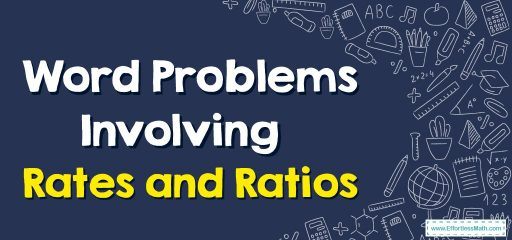# Word Problems Involving Rates and RatiosThe ratio is to compare two numbers.
Rate is one type of ratio and is used to measure the variety of one thing or quantity in comparison to other.
Word problems involving comparing rates deal with distances, time, rates, wind or water current, money, and age.

## A step-by-step guide to solving rates and ratios word problems

To solve the word problems involving rates and ratios, follow these steps:
Step 1: Find the known ratio and the unknown ratio.
Step 2: Write the proportion.
Step 3: Use cross-multiply and solve.
Step 4: Plug the result into the unknown ratio to check the answers.

If 11 apple pies cost $88, what will 8 apple pies cost? Solution: Write as a rate. $$\frac{88÷11}{11÷11}=\frac{8}{1}$$ Write a proportion to know the cost of 8 apple pies. $$\frac{8}{1}=\frac{x}{6}→8×6=1×x→x=48$$ ### Word Problems Involving Rates and Ratios – Examples 2 If 6 cookbooks cost$120, how much would a dozen cookbooks cost?
Solution:
Write as a rate. $$\frac{120÷6}{6÷6}=\frac{20}{1}$$
Write a proportion to know the cost of 12 cookbooks.
$$\frac{20}{1}=\frac{x}{12}→20×12=1×x→x=240$$

### What people say about "Word Problems Involving Rates and Ratios - Effortless Math: We Help Students Learn to LOVE Mathematics"?

No one replied yet.

X
30% OFF

Limited time only!

Save Over 30%

SAVE $5 It was$16.99 now it is \$11.99